Properties of Determinant

Chapter 4 Class 12 Determinants
Serial order wise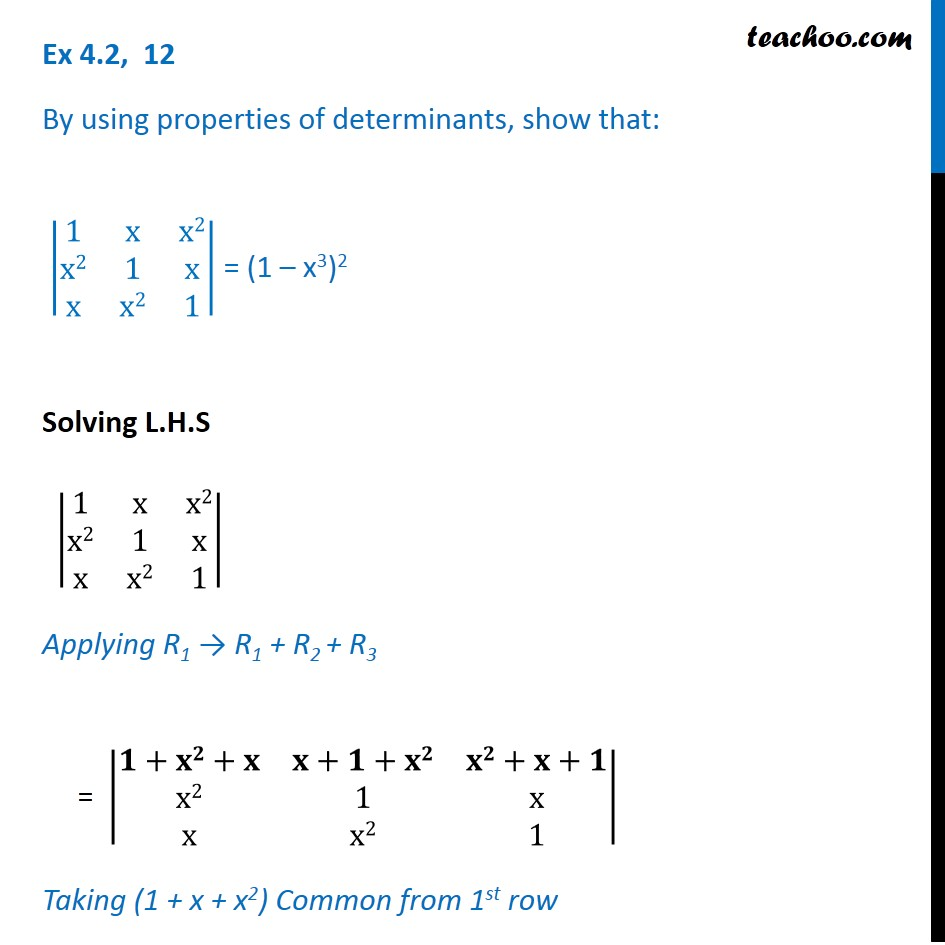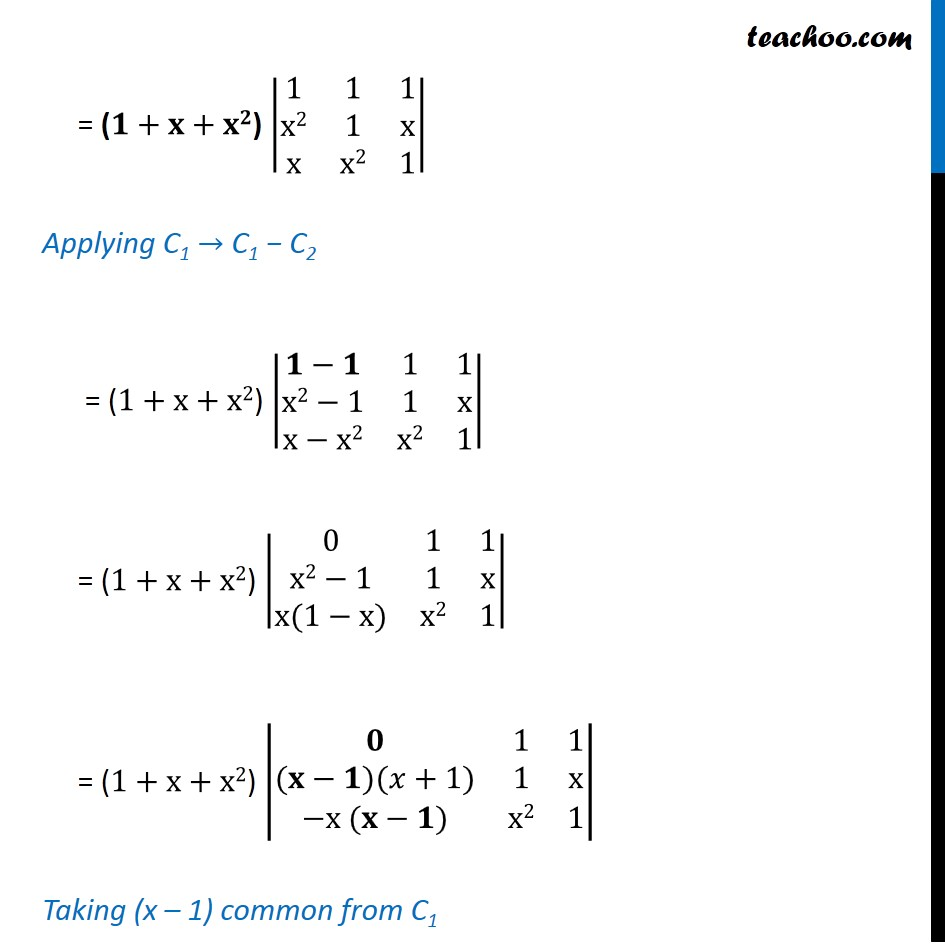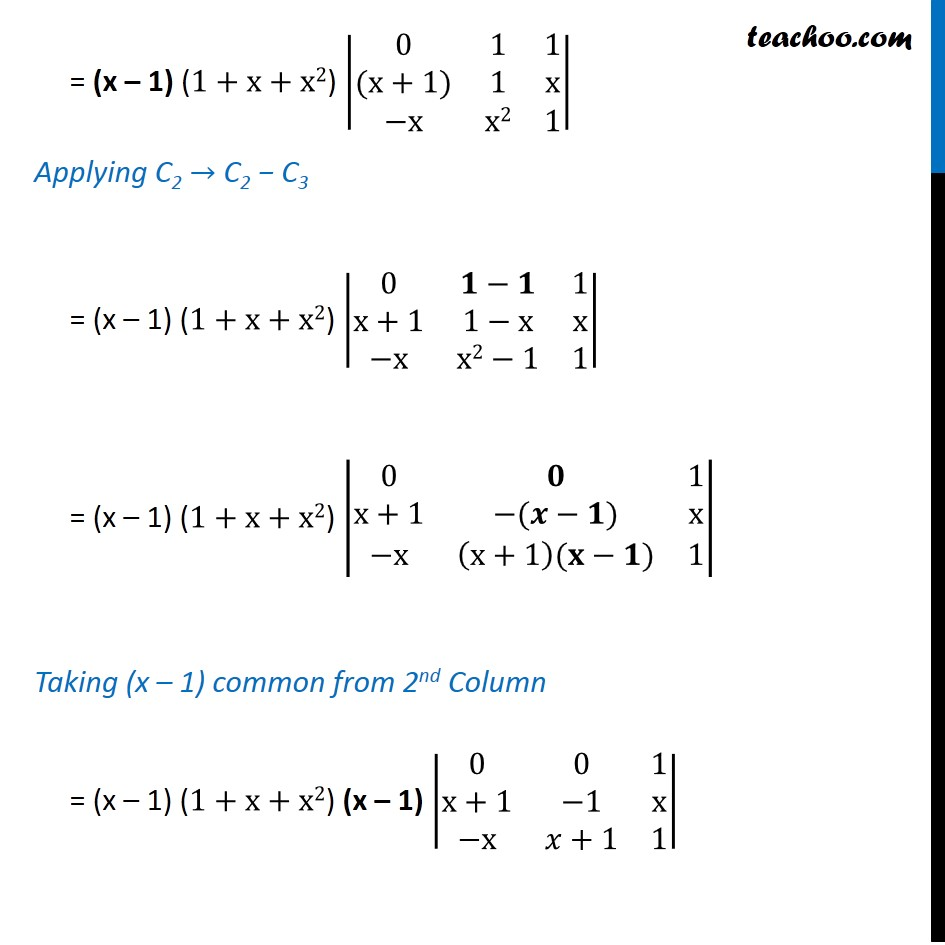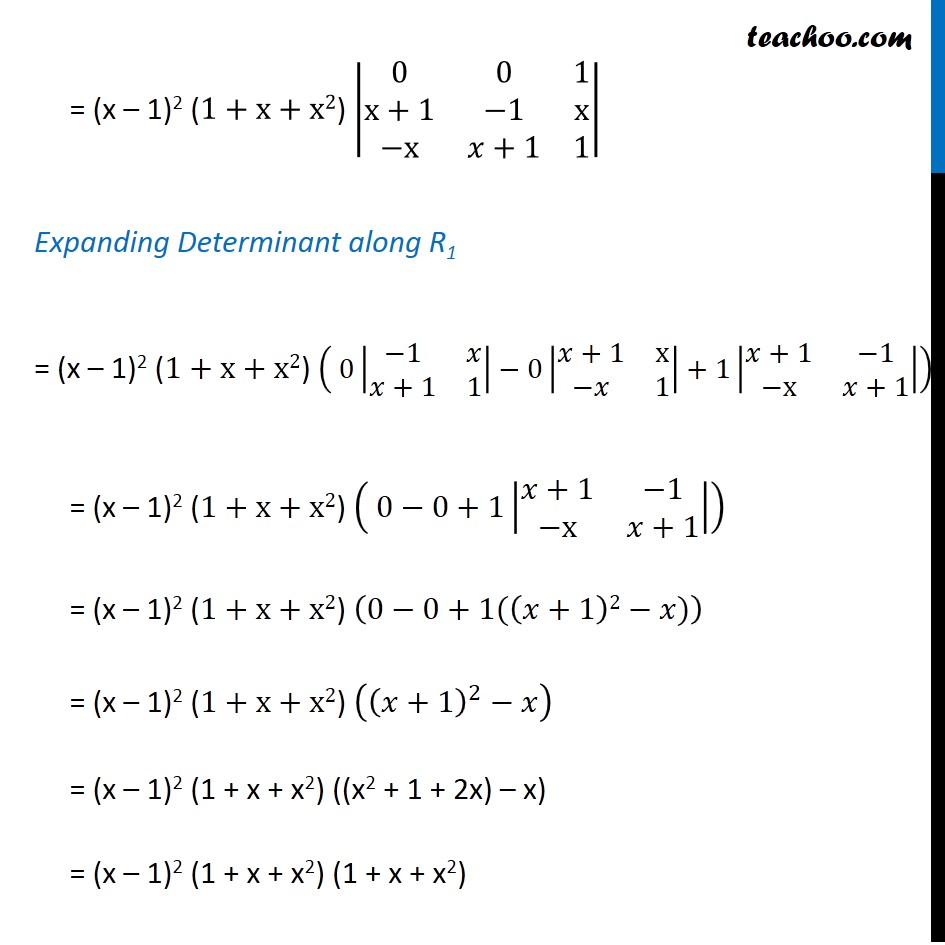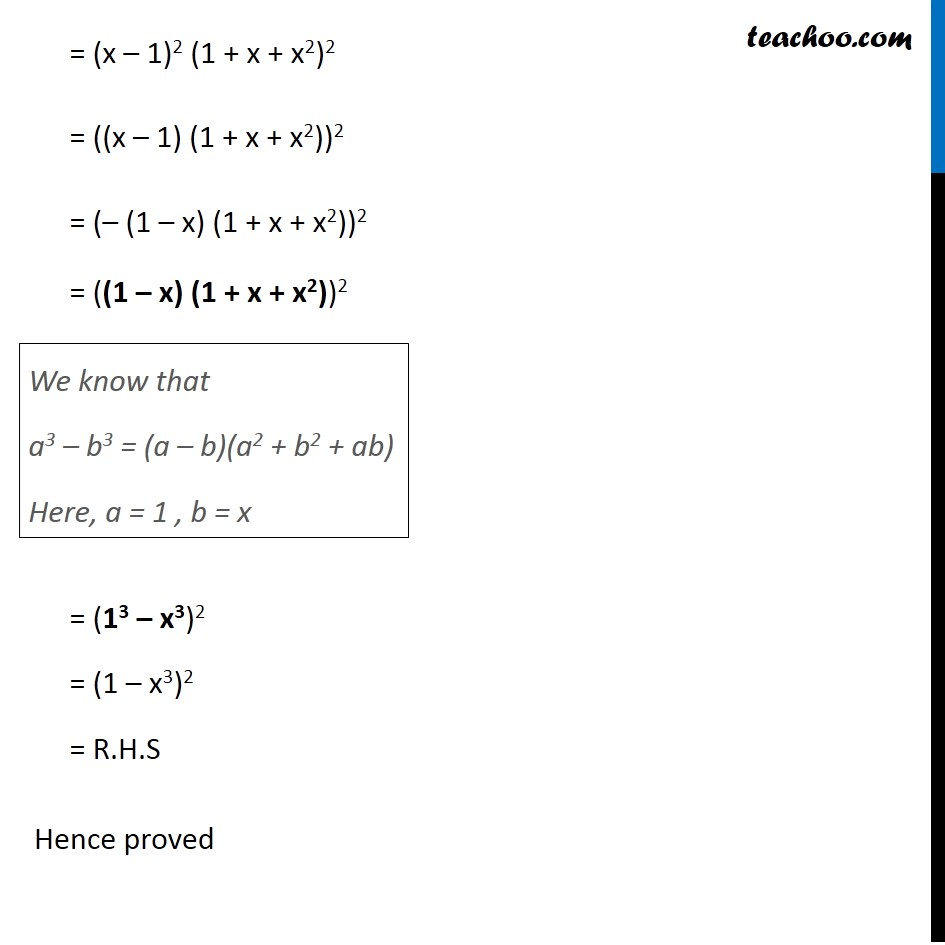Learn in your speed, with individual attention - Teachoo Maths 1-on-1 Class

### Transcript

Question 12 By using properties of determinants, show that: |■8(1&x&x2@x2&1&x@x&x2&1)| = (1 – x3)2 Solving L.H.S |■8(1&x&x2@x2&1&x@x&x2&1)| Applying R1 → R1 + R2 + R3 = |■8(𝟏+𝐱𝟐+𝐱&𝐱+𝟏+𝐱𝟐&𝐱𝟐+𝐱+𝟏@x2&1&x@x&x2&1)| Taking (1 + x + x2) Common from 1st row = (𝟏+𝐱+𝐱𝟐) |■8(1&1&1@x2&1&x@x&x2&1)| Applying C1 → C1 − C2 = (1+x+x2) |■8(𝟏−𝟏&1&1@x2−1&1&x@x−x2&x2&1)| = (1+x+x2) |■8(0&1&1@x2−1&1&x@x(1−x)&x2&1)| = (1+x+x2) |■8(𝟎&1&1@(𝐱−𝟏)(𝑥+1)&1&x@−x (𝐱−𝟏)&x2&1)| Taking (x – 1) common from C1 = (x – 1) (1+x+x2) |■8(0&1&1@(x+1)&1&x@−x&x2&1)| Applying C2 → C2 − C3 = (x – 1) (1+x+x2) |■8(0&𝟏−𝟏&1@x+1&1−x&x@−x&x2−1&1)| = (x – 1) (1+x+x2) |■8(0&𝟎&1@x+1&−(𝒙−𝟏)&x@−x&(x+1)(𝐱−𝟏)&1)| Taking (x – 1) common from 2nd Column = (x – 1) (1+x+x2) (x – 1) |■8(0&0&1@x+1&−1&x@−x&𝑥+1&1)| = (x – 1)2 (1+x+x2) |■8(0&0&1@x+1&−1&x@−x&𝑥+1&1)| Expanding Determinant along R1 = (x – 1)2 (1+x+x2) ( 0|■8(−1&𝑥@𝑥+1&1)|−0|■8(𝑥+1&x@−𝑥&1)|+1|■8(𝑥+1&−1@−x&𝑥+1)|) = (x – 1)2 (1+x+x2) ( 0−0+1|■8(𝑥+1&−1@−x&𝑥+1)|) = (x – 1)2 (1+x+x2) (0−0+1((𝑥+1)2−𝑥)) = (x – 1)2 (1+x+x2) ((𝑥+1)^2−𝑥) = (x – 1)2 (1 + x + x2) ((x2 + 1 + 2x) – x) = (x – 1)2 (1 + x + x2) (1 + x + x2) = (x – 1)2 (1 + x + x2)2 = ((x – 1) (1 + x + x2))2 = (– (1 – x) (1 + x + x2))2 = ((1 – x) (1 + x + x2))2 = (13 – x3)2 = (1 – x3)2 = R.H.S Hence proved = (x – 1)2 (1 + x + x2)2 = ((x – 1) (1 + x + x2))2 = (– (1 – x) (1 + x + x2))2 = ((1 – x) (1 + x + x2))2 = (13 – x3)2 = (1 – x3)2 = R.H.S Hence proved We know that a3 – b3 = (a – b)(a2 + b2 + ab) Here, a = 1 , b = x